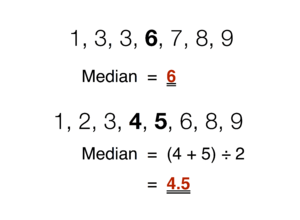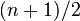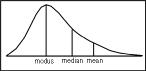Median facts for kids

Kids Encyclopedia FactsFinding the median in sets of data with an odd and even number of values.

In probability theory and statistics, the median is a number. This number has the property that it divides a set of observed values in two equal halves, so that half of the values are below it, and half are above.

If there are a finite number of elements, the median is easy to find. The values need to be arranged in a list, lowest to highest. If there is an odd number of values, the median is the one at position$(n+1)/2$. For example, if there are 13 values, they can be arranged into two groups of 6, with the median in between, at position 7. With an even number of values, as there is no single number which divides all of the numbers to two halves, the median is defined as the mean of the two central elements. With 14 observations, this would be the mean of elements 7 and 8, which is their sum divided by 2.

Alternatively, the median of an even-sized list is sometimes defined as either of the two middle elements; the choice being either (a) always the smallest one, (b) always the largest one, or (c) randomly choose between the two. This alternative definition has two important advantages: it guarantees that the median is always a list element (e.g., a list of integers will never have a fractional median), and it guarantees that the median exists for any ordinal-valued data. On the other hand, when one of the choices (a) or (b) is taken, the median of a sample will be biased, which is an unwanted property of a statistical estimator. Definition (c) does not have that disadvantage, but it is more difficult to do. It also has the disadvantage that the same list of values does not have a well-defined, deterministic median.Distribution curve with mode, median and mean. Median is the middle.

Median and mean

Median and mean are different in several ways. Mean is a better measure in many cases, because many of the statistical tests can use mean and standard deviation of two observations to compare them, while the same comparison cannot be performed using the medians.

Median is more useful when the variance of the values is not important and we only need a central measure of the values. If the maximum value of a set of numbers changes while the other numbers of this set are kept the same, the mean of this set of numbers changes, but the median does not.

Another advantage of median is that it can be calculated sooner when we are studying survival data. For example, a researcher can calculate the median survival of patients with a kidney transplant, when half the patients participated in his study die. Calculate the mean survival requires continuing the study and following all the patients until their death.

Example

Suppose you want to know how many jelly beans most people in the room have. Let's say there are five people in the room:

• Person 1 (7 jelly beans), Person 2 (8 jelly beans), Person 3 (9 jelly beans), Person 4 (10 jelly beans), Person 5 (11 jelly beans)
• To calculate the mean you would add the total number of jelly beans (45) and divide by the number of people (5): the mean is 9 jelly beans per person.
• To calculate the median, you line up amounts of jelly beans (7, 8, 9, 10, 11), and choose the middle number: the median is also 9 jelly beans.

The results change a lot when you have a bigger range of numbers. Let's imagine a different group of five people:

• Person 1 (8 jelly beans), Person 2 (8 jelly beans), Person 3 (9 jelly beans), Person 4 (10 jelly beans), Person 5 (50 jelly beans)
• To calculate the mean you would add the total number of jelly beans (85) and divide by the number of people (5): the mean is now 17 jelly beans per person.
• To calculate the median, you line up amounts of jelly beans (8, 8, 9, 10, 50), and choose the middle number: the median is still 9 jelly beans.

In this second case, the mean gives you a poor understanding how many jelly beans most people have (10 or less). The median gives you a better idea of the number of jelly beans most people have. If you wanted to divide up the number of jelly beans equally, however, you would use the mean. The median is basically the middle number.Median Facts for Kids. Kiddle Encyclopedia.